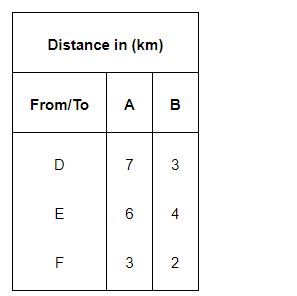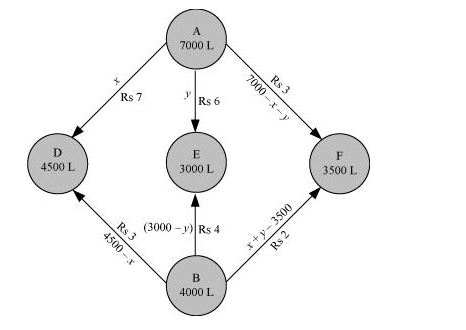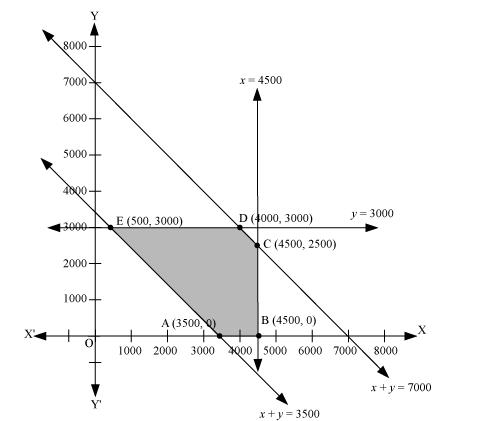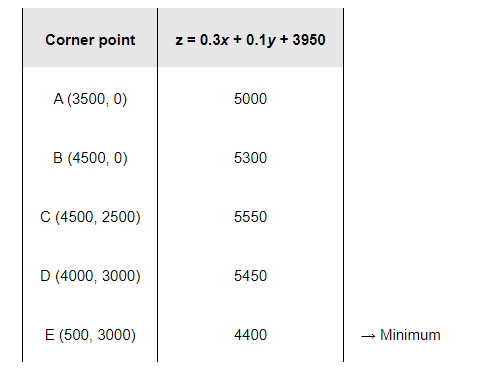# An oil company has two depots A and B with capacities of 7000 L and 4000 L respectively.

Question:

An oil company has two depots A and B with capacities of 7000 L and 4000 L respectively. The company is to supply oil to three petrol pumps, D, E and F whose requirements are 4500L, 3000L and 3500L respectively. The distance (in km) between the depots and the petrol pumps is given in the following table:Assuming that the transportation cost of 10 litres of oil is Re 1 per km, how should the delivery be scheduled in order that the transportation cost is minimum? What is the minimum cost?

Solution:

Let x and y litres of oil be supplied from A to the petrol pumps, D and E. Then, (7000 − x − y) will be supplied from A to petrol pump F.

The requirement at petrol pump D is 4500 L. Since x L are transported from depot A, the remaining (4500 −x) L will be transported from petrol pump B.

Similarly, (3000 − y) L and 3500 − (7000 − x − y) = (x + y − 3500) L will be transported from depot B to petrol pump E and F respectively.

The given problem can be represented diagrammatically as follows.$x \geq 0, y \geq 0$, and $(7000-x-y) \geq 0$

$\Rightarrow x \geq 0, y \geq 0$, and $x+y \leq 7000$

$4500-x \geq 0,3000-y \geq 0$, and $x+y-3500 \geq 0$

$\Rightarrow x \leq 4500, y \leq 3000$, and $x+y \geq 3500$

Cost of transporting 10 L of petrol = Re 1

Cost of transporting $1 \mathrm{~L}$ of petrol $=\mathrm{Rs} \frac{1}{10}$

Therefore, total transportation cost is given by,

$z=\frac{7}{10} \times x+\frac{6}{10} y+\frac{3}{10}(7000-x-y)+\frac{3}{10}(4500-x)+\frac{4}{10}(3000-y)+\frac{2}{10}(x+y-3500)$

$=0.3 x+0.1 y+3950$

The problem can be formulated as follows.

Minimize $z=0.3 x+0.1 y+3950$             (1)

subject to the constraints,

$x+y \leq 7000$                                      (2)

$x \leq 4500$                                          (3)

$y \leq 3000$                                         (4)

$x+y \geq 3500$                                    (5)

$x, y \geq 0$                                          (6)

The feasible region determined by the constraints is as follows.The corner points of the feasible region are A (3500, 0), B (4500, 0), C (4500, 2500), D (4000, 3000), and E (500, 3000).

The values of z at these corner points are as follows.The minimum value of z is 4400 at (500, 3000).

Thus, the oil supplied from depot A is 500 L, 3000 L, and 3500 L and from depot B is 4000 L, 0 L, and 0 L to petrol pumps D, E, and F respectively.

The minimum transportation cost is Rs 4400.